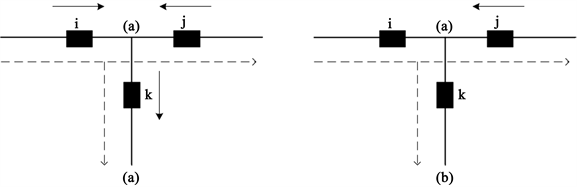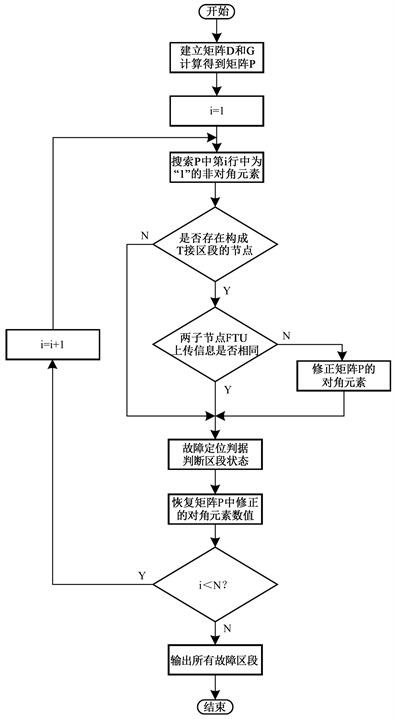#### 期刊菜单

Fault Location of Distribution Network with Distributed Generation Based on Improved Matrix Algorithm
DOI: 10.12677/SG.2019.96025, PDF, HTML, XML, 下载: 691  浏览: 2,029

Abstract: The access of distributed generation (DG) puts forward higher requirements for fault location of distribution network. In order to solve the problem that the T-wiring segment in the distribution network with DG may lead to the misjudgment of the matrix algorithm fault location, the traditional matrix algorithm is improved. Firstly, based on the topology connection relationship of the distribution network, and the feeder terminal unit (FTU) uploading fault overcurrent information, the network description matrix and the fault information matrix are constructed, and the fault judgment matrix is calculated. Secondly, if the FTU uploading information of the two sub-nodes of the T-wiring segment in the distribution network is different, the fault judgment matrix is corrected; finally, the fault section is located by using the fault location criterion. The results of the example show that the proposed method can locate fault sections accurately when single and multiple faults occur in the case of T-wiring segment in the distribution network with DG.

1. 引言

2. 基于传统矩阵算法的配电网故障定位基本原理

2.1. FTU上传信息机制

2.2. 网络描述矩阵D

${d}_{ij}=\left\{\begin{array}{l}1节点i与j分别为某区段的父、子节点\\ 0其他情况\end{array}$ (1)

2.3. 故障信息矩阵G

${g}_{ii}=\left\{\begin{array}{l}-1\text{ }节点i处\text{FTU}检测到过电流且方向与全网正方向相反\\ 0节点i处\text{FTU}未检测到过电流\\ 1\text{ }节点i处\text{FTU}检测到过电流且方向与全网正方向相同\end{array}$ (2)

2.4. 故障判断矩阵P

2.5. 故障定位判据

1) 配电网发生单重故障：若 ${p}_{ii}=1$，所有的 ${p}_{ij}=1$ ( $i\ne j$ )，有 ${p}_{jj}=0$ 或−1

2) 配电网发生多重故障：若 ${p}_{ii}=0$，所有的 ${p}_{ij}=1$ ( $i\ne j$ )，有 ${p}_{jj}=-1$

1) 配电网发生单重故障：若 ${p}_{ii}=1$，所有的 ${p}_{ij}=0$ ( $i\ne j$ )

3. 基于改进矩阵算法的配电网故障定位基本原理

3.1. T接区段对故障定位的影响Figure 1. Simplified diagram of T-wiring segment of distribution network with DG

3.2. 改进矩阵算法基本原理

1) 通过故障判断矩阵每行的非对角元素，判断哪些节点共同构成T接区段；

2) 通过故障判断矩阵中每行的对角元素，判断T接区段两个子节点FTU上传信息是否相同；

3) 若T接区段两个子节点FTU上传信息不同，则利用等效的思想，在满足T接区段状态不变的前提下，将两个子节点FTU上传信息修正使二者相同，即修正对应的故障判断矩阵对角元素。修正原则为：若两个子节点FTU上传信息不同，只要有一个为“1”，二者都修正为“1”；若没有“1”，只要有一个“−1”，二者都修正为“−1”；

4) 对故障判断矩阵修正后，利用矩阵算法故障定位判据，判断该T接区段是否为故障区段；该T接区段判断结束后，为了避免影响其他区段定位结果，故障判断矩阵修正的对角元素恢复原值。

3.3. 改进矩阵算法故障定位流程Figure 2. Flow chart of fault location of distribution network with DG

4. 算例分析Figure 3. Simplified diagram of distribution network with DG

$D=\left[\begin{array}{cccccccccc}0& 1& 0& 0& 0& 0& 0& 0& 0& 0\\ 0& 0& 1& 0& 1& 0& 0& 0& 0& 0\\ 0& 0& 0& 1& 0& 0& 0& 0& 0& 0\\ 0& 0& 0& 0& 0& 0& 0& 0& 0& 0\\ 0& 0& 0& 0& 0& 1& 1& 0& 0& 0\\ 0& 0& 0& 0& 0& 0& 0& 0& 0& 0\\ 0& 0& 0& 0& 0& 0& 0& 1& 1& 0\\ 0& 0& 0& 0& 0& 0& 0& 0& 0& 0\\ 0& 0& 0& 0& 0& 0& 0& 0& 0& 1\\ 0& 0& 0& 0& 0& 0& 0& 0& 0& 0\end{array}\right]$ (3)

$G=\left[\begin{array}{cccccccccc}1& 0& 0& 0& 0& 0& 0& 0& 0& 0\\ 0& 1& 0& 0& 0& 0& 0& 0& 0& 0\\ 0& 0& 0& 0& 0& 0& 0& 0& 0& 0\\ 0& 0& 0& 0& 0& 0& 0& 0& 0& 0\\ 0& 0& 0& 0& 1& 0& 0& 0& 0& 0\\ 0& 0& 0& 0& 0& 0& 0& 0& 0& 0\\ 0& 0& 0& 0& 0& 0& -1& 0& 0& 0\\ 0& 0& 0& 0& 0& 0& 0& -1& 0& 0\\ 0& 0& 0& 0& 0& 0& 0& 0& -1& 0\\ 0& 0& 0& 0& 0& 0& 0& 0& 0& -1\end{array}\right]$ (4)

$P=D+G=\left[\begin{array}{cccccccccc}1& 1& 0& 0& 0& 0& 0& 0& 0& 0\\ 0& 1& 1& 0& 1& 0& 0& 0& 0& 0\\ 0& 0& 0& 1& 0& 0& 0& 0& 0& 0\\ 0& 0& 0& 0& 0& 0& 0& 0& 0& 0\\ 0& 0& 0& 0& 1& 1& 1& 0& 0& 0\\ 0& 0& 0& 0& 0& 0& 0& 0& 0& 0\\ 0& 0& 0& 0& 0& 0& -1& 1& 1& 0\\ 0& 0& 0& 0& 0& 0& 0& -1& 0& 0\\ 0& 0& 0& 0& 0& 0& 0& 0& -1& 1\\ 0& 0& 0& 0& 0& 0& 0& 0& 0& -1\end{array}\right]$ (5)

1) ${p}_{11}=1$${p}_{12}=1$${p}_{22}=1$，节点1,2构成的双端馈线区段(1)正常；

2) ${p}_{22}=1$${p}_{23}={p}_{25}=1$，节点2,3,5构成T接区段(2)；

${p}_{22}=1$${p}_{23}=1$${p}_{33}=0$，T接区段(2)故障；

${p}_{22}=1$${p}_{25}=1$${p}_{55}=1$，T接区段(2)正常；综上考虑，可得T接区段(2)错判。

3) ${p}_{33}=0$${p}_{34}=1$${p}_{44}=0$，节点3,4构成的双端馈线区段(3)正常；

4) ${p}_{55}=1$${p}_{56}={p}_{57}=1$，节点5,6,7构成T接区段(5)；

${p}_{55}=1$${p}_{56}=1$${p}_{66}=0$，T接区段(5)故障；

${p}_{55}=1$${p}_{57}=1$${p}_{77}=-1$，T接区段(5)故障；综上考虑，可得T接区段(5)故障。

1) ${p}_{22}=1$${p}_{23}={p}_{25}=1$，节点2,3,5构成T接区段(2)；

${p}_{33}=0$${p}_{55}=1$，两个子节点3,5处FTU上传信息不同；

${p}_{33}={p}_{55}=1$，两个子节点3,5处FTU上传信息修正；

${p}_{22}=1$${p}_{23}=1$${p}_{33}=1$，T接区段(2)正常；

${p}_{22}=1$${p}_{25}=1$${p}_{55}=1$，T接区段(2)正常；综上考虑，可得T接区段(2)正常。

2) ${p}_{55}=1$${p}_{56}={p}_{57}=1$，节点5,6,7构成T接区段(5)；

${p}_{66}=0$${p}_{77}=-1$，两个子节点6,7处FTU上传信息不同；

${p}_{66}={p}_{55}=-1$，两个子节点6,7处FTU上传信息修正；

${p}_{55}=1$${p}_{56}=1$${p}_{66}=-1$，T接区段(5)故障；

${p}_{55}=1$${p}_{57}=1$${p}_{77}=-1$，T接区段(5)故障；综上考虑，可得T接区段(5)故障。Table 1. Fault location simulation result

5. 结语

  马士聪, 高厚磊, 徐丙垠, 等. 配电网故障定位技术综述[J]. 电力系统保护与控制, 2009, 37(11): 119-124.  唐金锐, 尹项根, 张哲, 等. 配电网故障自动定位技术研究综述[J]. 电力自动化设备, 2013, 33(5): 7-13.  刘健, 张小庆, 同向前, 等. 含分布式电源配电网的故障定位[J]. 电力系统自动化, 2013, 37(2): 36-42.  孙景钌, 陈荣柱, 蔡轼, 等. 含分布式电源配电网的故障定位新方案[J]. 电网技术, 2013, 37(6): 1645-1650.  梅念, 石东源, 杨增力, 等. 一种实用的复杂配电网故障定位的矩阵算法[J]. 电力系统自动化, 2007, 31(10): 66-70.  刘健, 倪建立, 杜宇. 配电网故障区段判断和隔离的统一矩阵算法[J]. 电力系统自动化, 1999(1): 31-33.  卫志农, 何桦, 郑玉平. 配电网故障定位的一种新算法[J]. 电力系统自动化, 2001, 25(14): 48-50.  蒋秀洁, 熊信银, 吴耀武, 等. 改进矩阵算法及其在配电网故障定位中的应用[J]. 电网技术, 2004(19): 63-66.  马强, 张利民, 刘皓明. 配电网故障区间判断的通用矩阵算法[J]. 电力系统保护与控制, 2009(5): 22-25.  罗梅, 杨洪耕. 配电网故障定位的一种改进通用矩阵算法[J]. 电力系统保护与控制, 2012, 40(5): 64-68.